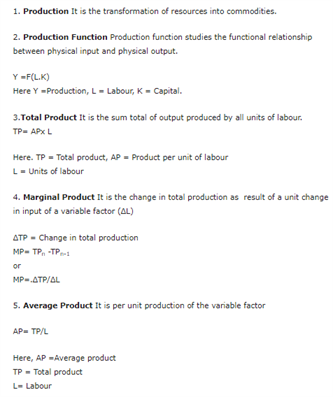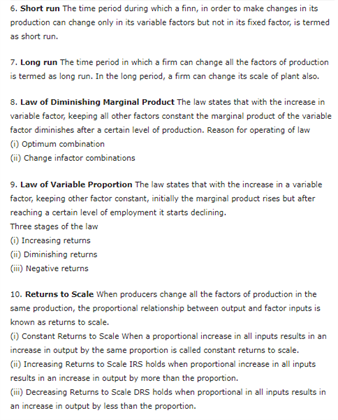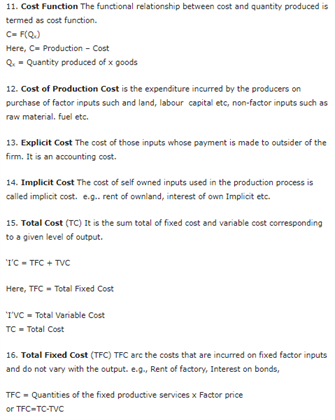# Revision Notes For Class 12 Economics Microeconomics Chapter 3 Production And Costs

Production And Costs is the chapter that explains about the pricing structure for a commodity. This chapter is incorporated with various topics – production, production function, total product (TP), marginal product (MP), average product (AP), short run, long run, law of diminishing marginal product, law of variable proportion, returns to scale, cost function, production cost, explicit cost, implicit cost, total cost, total fixed cost, total variable cost, average cost, average variable cost, marginal cost, shape of curves.